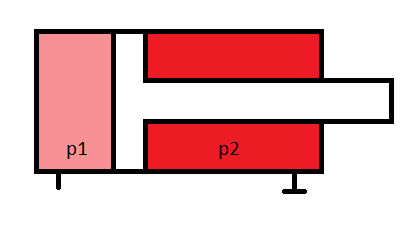Pressure Multiplication

As we read in the previous article ‘How Hydraulic works: Basics Part-I‘ we knew how force is multiplying in hydraulic system. But its reverse is similarly correct. In other words we can multiply pressure according to our use i.e Pressure Multiplication.

Sometimes for industrial use we have to increase the pressure without increasing the force, like high pressure pump for fuel injection. Then we can use this method for increasing pressure with the help of low pressure.

We know that Pressure,

p = F/A

Let the force ‘F’ acts over an area ‘A1’ and then reduce the area by ‘A2’ it will increase the pressure or we can say we multiplication of pressure.

Let, p1 = 10 bar (Low pressure available)

Note: bar is the unit of pressure usually use in industrial application.

1 bar = 100000 N/m2

A1 = 100 cm2

A2 = 1 cm2

F1 = p1 X A1

F1 = 10 x 100 = 1000

Same force will act on area A2,

p2 = F/A2

p2 = 1000/1 = 1000 bar.

Hence, we multiplied the pressure 100 times with the available pressure. Similarly like we multiply the force.

Pressure multiplication in double acting cylinder.Fig. 2 pressure multiplication in double acting hydraulic cylinder.

Here is one of the reason for cylinder seal damage. As per the pressure multiplication theory you know even there is low pressure in larger area (piston end) pressure may be much higher in other end of the piston (rod end) due to less area available.

Now, suppose if system pressure for preventing the damage of cylinder seal is 250 bar. This mean maximum pressure should not increase 250 bar ever. But due to some reason your rod end is block and now hydraulic oil is not flowing from rod end. If you have set pressure relief valve on 250 bar and at your piston end you have maximum 250 bar then definitely due to pressure multiplication, the rod end pressure will much higher then 250 and ultimately damage the seal.

In conclusion, if we are using pressure multiplication according to our purpose then it is beneficial but however it has negative effects too. So, be care full and must consider this effects while setting maximum pressure of system.

This pressure multiplication is also known as Pressure Intensification.

Hope you enjoyed this article. Please do share and support.

Till then, Good Bye.

1 thought on “How Hydraulic works: Basics Part-II”

1.Daphne Gilpin says:

Thanks for explaining that sometimes it’s necessary to increase the pressure without increasing the force in industrial hydraulic applications. I’ve been trying to learn more about how hydraulics work because the company I work for needs to buy some seals for our new machine. I’m glad I read your article because it was interesting to learn about the different combinations of pressure and force used in hydraulic cylinder seals.